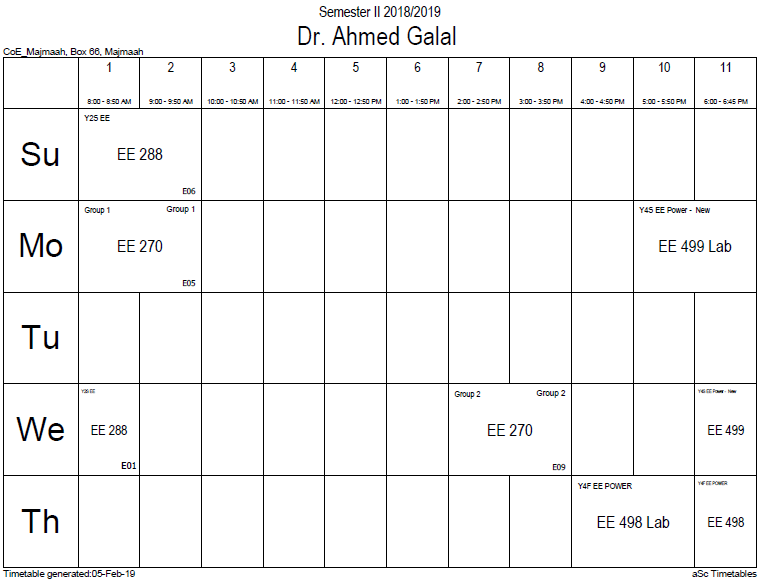## P and E in various

In a homogeneous linear and isotropic dielectric medium, the polarization is aligned with and proportional to the electric field E:${mathbf P}=varepsilon_0chi{mathbf E},$

where ε0 is the electric constant, and χ is the electric susceptibility of the medium.

In an anisotropic material, the polarization and the field are not necessarily in the same direction. Then, the ith component of the polarization is related to the jth component of the electric field according to:$P_i = sum_j epsilon_0 chi_{ij} E_j , ,!$

where ε0 is the electric constant, and χij, is the electric susceptibility tensor of the medium. This relation shows, for example, that a material can polarize in the x direction by applying a field in the z direction, and so on. The case of an anisotropic dielectric medium is described by the field of crystal optics.

As in most electromagnetism, this relation deals with macroscopic averages of the fields and dipole density, so that one has a continuum approximation of the dielectric materials that neglects atomic-scale behaviors. The polarizability of individual particles in the medium can be related to the average susceptibility and polarization density by the Clausius-Mossotti relation.

In general, the susceptibility is a function of the frequency ω of the applied field. When the field is an arbitrary function of time t, the polarization is a convolution of the Fourier transform of χ(ω) with the E(t). This reflects the fact that the dipoles in the material cannot respond instantaneously to the applied field, and causality considerations lead to the Kramers–Kronig relations.

If the polarization P is not linearly proportional to the electric field E, the medium is termed nonlinear and is described by the field of nonlinear optics. To a good approximation (for sufficiently weak fields, assuming no permanent dipole moments are present), P is usually given by a Taylor series in E whose coefficients are the nonlinear susceptibilities:$P_i / epsilon_0 = sum_j chi^{(1)}_{ij} E_j + sum_{jk} chi_{ijk}^{(2)} E_j E_k + sum_{jkell} chi_{ijkell}^{(3)} E_j E_k E_ell + cdots !$

where$chi^{(1)}$ is the linear susceptibility,$chi^{(2)}$ is the second-order susceptibility (describing phenomena such as the Pockels effect, optical rectification and second-harmonic generation), and$chi^{(3)}$ is the third-order susceptibility (describing third-order effects such as the Kerr effect and electric field-induced optical rectification).

In ferroelectric materials, there is no one-to-one correspondence between P and E at all because of hysteresis.

### Office HoursNo office hours### My Timetable### Contactsemail: [email protected]

Phone: 2570

### Welcome

Welcome To Faculty of Engineering### IEEEhttp://www.ieee.org/

/

### Bookmarkshttp://www.utk.edu/research/

http://science.doe.gov/grants/index.asp

http://www1.eere.energy.gov/vehiclesandfuels/

http://www.eere.energy.gov/

### Upcoming Conferences### Engineering quotes### Travel Web Siteshttp://www.hotels.com/

http://www.orbitz.com/

http://www.hotwire.com/us/index.jsp

http://www.kayak.com/

### Blackboardستقام اختبارات الميدتيرم يوم الثلاثاء 26-6-1440

حسب الجدول المعلن بلوحات الاعلان

### Summer trainingThe registration for summer training will start from 5th week of second semesterClass registration week 1

### برنامج التجسير### إحصائية الموقع

عدد الصفحات: 2879

البحوث والمحاضرات: 1280

الزيارات: 101088